# Robust Re-Identification by Multiple Views Knowledge Distillation

## VKD

Posted by JoselynZhao on December 14, 2020

# Introducation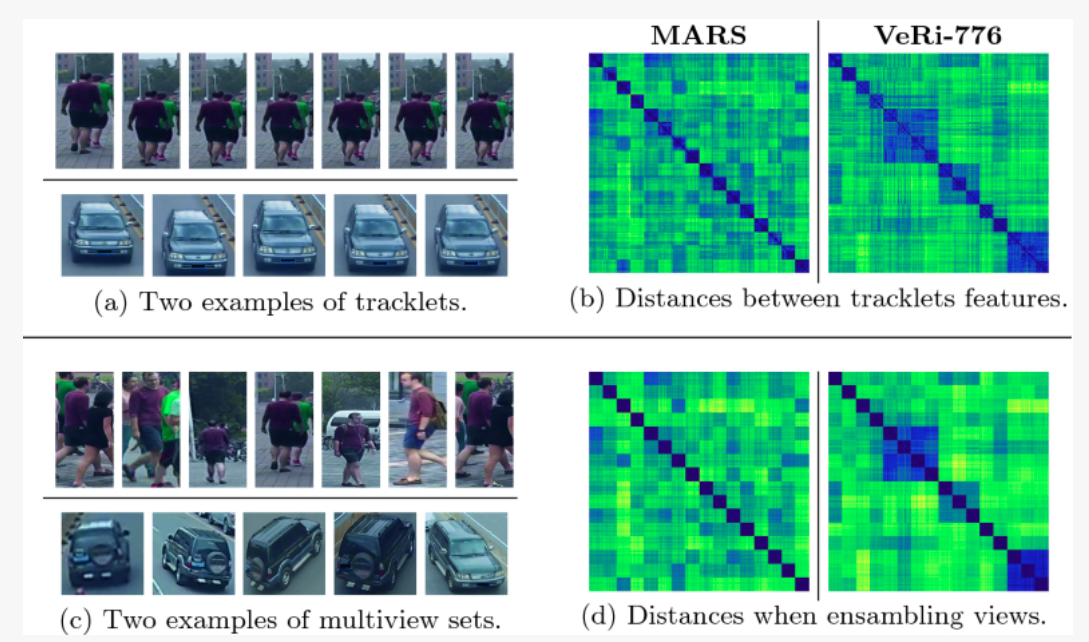## 动机

V2V he I2V之间还存在较大的差距。

As observed in , a large gap in Re-ID performance still subsists between V2V and I2V,

## VKD

we propose Views Knowledge Distillation (VKD), which transfers the knowledge lying in several views in a teacher-student fashion. VKD devises a two-stage procedure, which pins the visual variety as a teaching signal for a student who has to recover it using fewer views.

## 主要贡献

• i）学生的表现大大超过其老师，尤其是在“图像到视频”设置中；
• ii）彻底的调查显示，与老师相比，学生将更多的精力放在目标上，并且丢弃了无用的细节；
• iii）重要的是，我们不将分析局限于单个领域，而是在人，车辆和动物的Re-ID方面取得了出色的结果。
• i) the student outperforms its teacher by a large margin, especially in the Image-To-Video setting;
• ii) a thorough investigation shows that the student focuses more on the target compared to its teacher and discards uninformative details;
• iii) importantly, we do not limit our analysis to a single domain, but instead achieve strong results on Person, Vehicle and Animal Re-ID.

# Related works

• Image-To-Video Re-Identification.
• Knowledge Distillation

# Method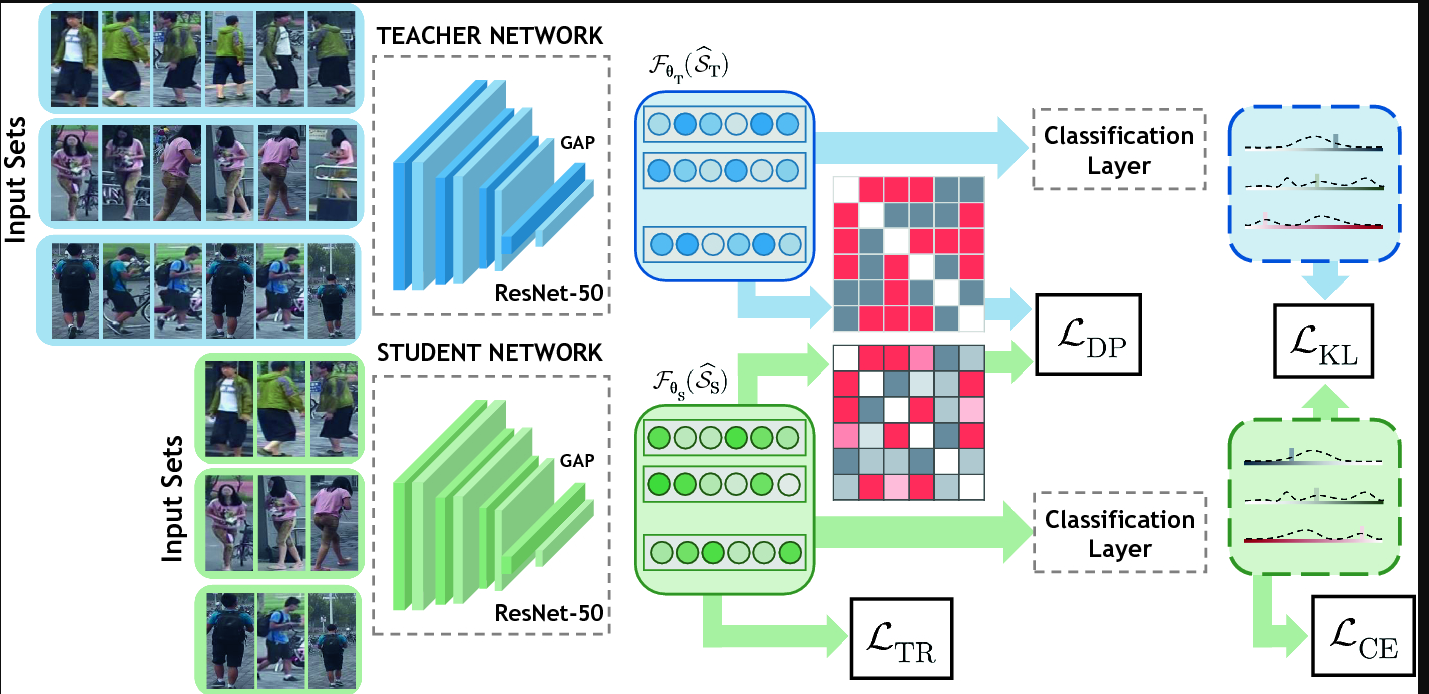图２VKD概述。学生网络被优化来在仅使用少量视图的情况下模仿老师的行为。

our proposal frames the training algorithm as a two-stage procedure, as follows

• First step (Sect. 3.1): the backbone network is trained for the standard Video-To-Video setting.
• Second step (Sect. 3.2): we appoint it as the teacher and freeze its parameters. Then, a new network with the role of the student is instantiated. As depicted in Fig. 2, we feed frames representing different views as input to the teacher and ask the student to mimic the same outputs from fewer frames.

第一步，用标准的Ｖ2V设置训练骨干网络。　 第二步，固定老师网络的参数，初始化学生网络。如图２所示，我们将表达不同视图的帧喂给老师网络，并且叫学生网络根据少量的帧来模仿相同的输出。

## Teacher Network

### Set Representation.

Here, we naively compute the set-level embedding $$\mathcal{F}(\mathcal{S})$$ through a temporal average pooling. While we acknowledge better aggregation modules exist, we do not place our focus on devising a new one, but instead on improving the earlier features extractor.

### Teacher Optimisation.

We train the base network - which will be the teacher during the following stage - combining a classification term $$\mathcal{L}_{CE}$$ (cross-entropy) with the triplet loss $$\mathcal{L}_{TR}$$ , The first can be formulated as: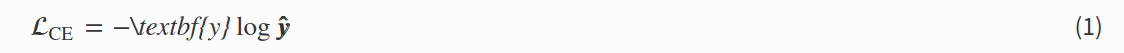其中 \textbf{y} 和$$\hat{\textbf{y}}$$ 分别表示one-shot 标签和softmax输出的标签。 $$\mathcal{L}_{TR}$$ 鼓励特征空间中的距离约束，将相同目标变得更近，不同目标变得更远。形式化为：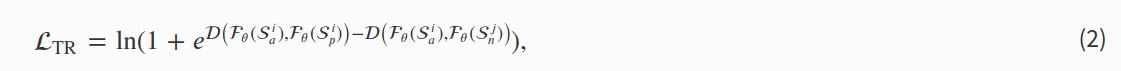其中，$$\mathcal{S}_p$$ 和$$\mathcal{S}_n$$分别为锚点$$\mathcal{S}_a$$在batch内的最强正锚点和负锚点。

## Views Knowledge Distillation (VKD)

Views Knowledge Distillation（VKD）通过迫使学生网络$$\mathcal{F}_{\theta_S}(\cdot)$$来匹配教师网络 $$\mathcal{F}_{\theta_T}(\cdot)$$的输出来解决问题。 为此，我们１）允许教师网络从不同的视角访问帧 $$\hat{S}_T = (\hat{s}_1,\hat{s}_2,\hat{s}_3,...,\hat{s}_N)$$，２）强迫学生网络根据 $$\hat{S}_S = (\hat{s}_1,\hat{s}_2,\hat{s}_3,...,\hat{s}_M)$$　来模仿教师网络的输出。其中候选量Ｍ<N (在文章实验中，Ｍ＝２，Ｎ＝８)．

Views Knowledge Distillation (VKD) stresses this idea by forcing a student network $$\mathcal{F}_{\theta_S}(\cdot)$$ to match the outputs of the teacher $$\mathcal{F}_{\theta_T}(\cdot)$$ . In doing so, we: i) allow the teacher to access frames $$\hat{S}_T = (\hat{s}_1,\hat{s}_2,\hat{s}_3,...,\hat{s}_N)$$ from different viewpoints; ii) force the student to mimic the teacher output starting from a subset $$\hat{S}_S = (\hat{s}_1,\hat{s}_2,\hat{s}_3,...,\hat{s}_M)$$with cardinality 𝑀<𝑁 (in our experiments, 𝑀=2 and 𝑁=8 ). The frames in $$\hat{S}_T$$ are uniformly sampled from $$\hat{S}_S$$ without replacement. This asymmetry between the teacher and the student leads to a self-distillation objective, where the latter can achieve better solutions despite inheriting the same architecture of the former.

VKD探索知识蒸馏损失为：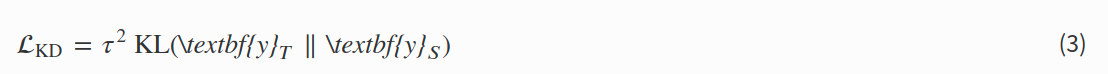In addition to fitting the output distribution of the teacher (Eq. 3), our proposal devises additional constraints on the embedding space learnt by the student. In details, VKD encourages the student to mirror the pairwise distances spanned by the teacher. Indicating with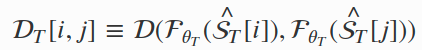he distance induced by the teacher between the i-th and j-th sets (the same notation $$\mathcal{D}_S[i,j]$$ also holds for the student), VKD seeks to minimise: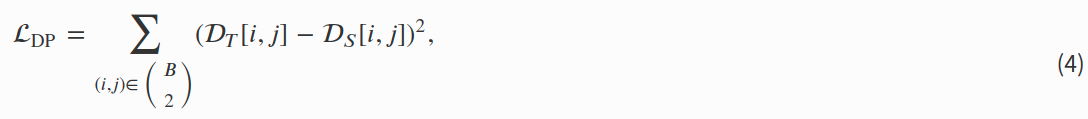where B equals the batch size.

### Student Optimisation.

The VKD overall objective combines the distillation terms ( $$\mathcal{L}_{KD}$$ and $$\mathcal{L}_{DP}$$ ) with the ones optimised by the teacher - $$\mathcal{L}_{CE}$$ and $$\mathcal{L}_{TR}$$ - that promote higher conditional likelihood w.r.t. ground truth labels. To sum up, VKD aims at strengthening the features of a CNN in Re-ID settings through the following optimisation problem: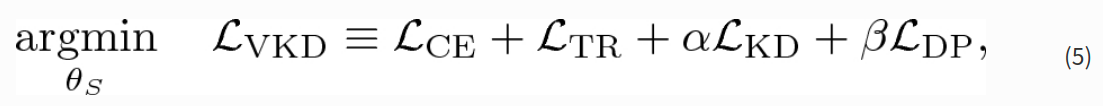# Experience

## 数据集

### Person Re-ID

• MARS
• Duke-Video-ReID

• VeRi-776

• Amur Tiger

## Self-distillation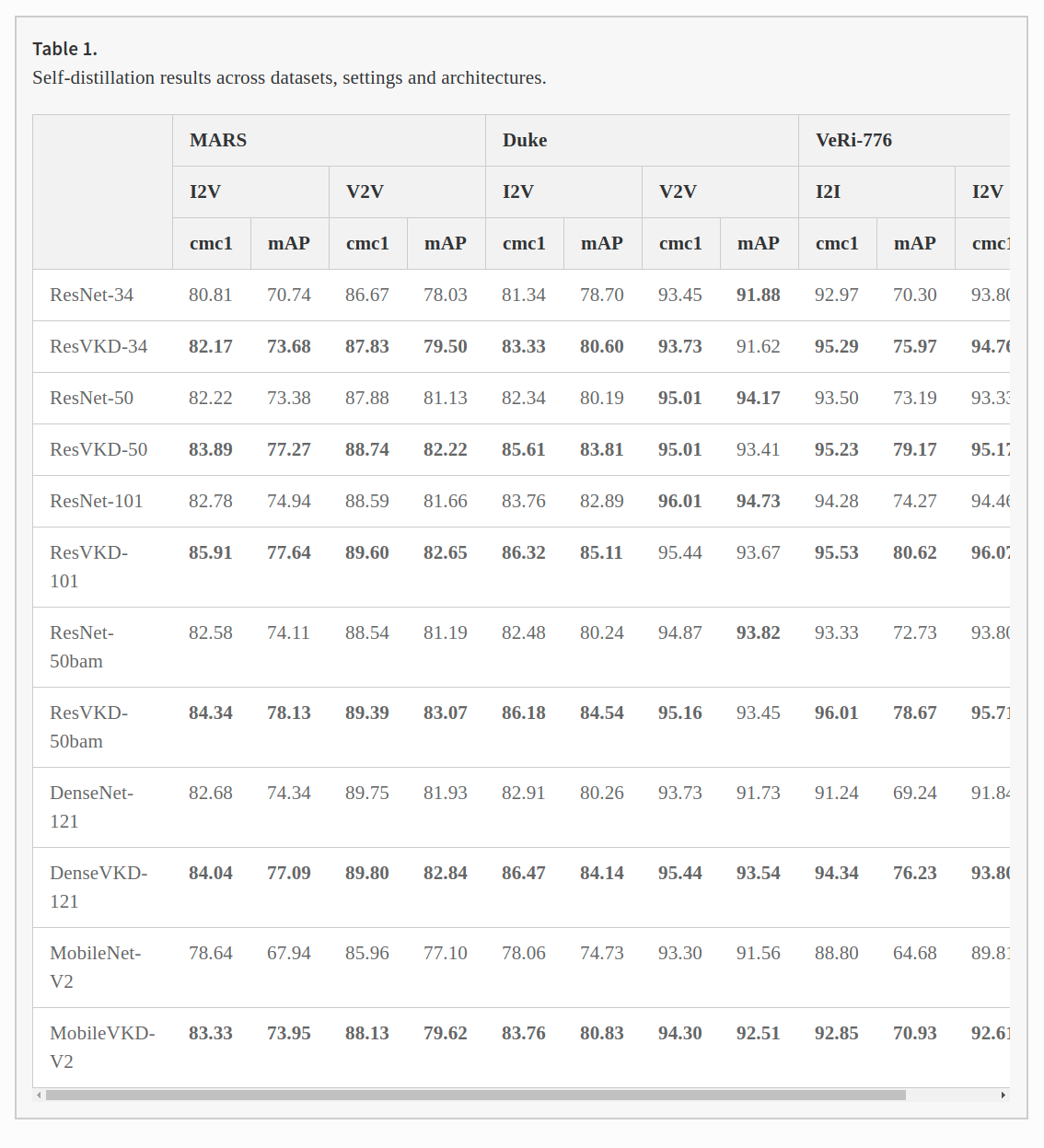Table 1 reports the comparisons for different backbones: in the vast majority of the settings, the student outperforms its teacher.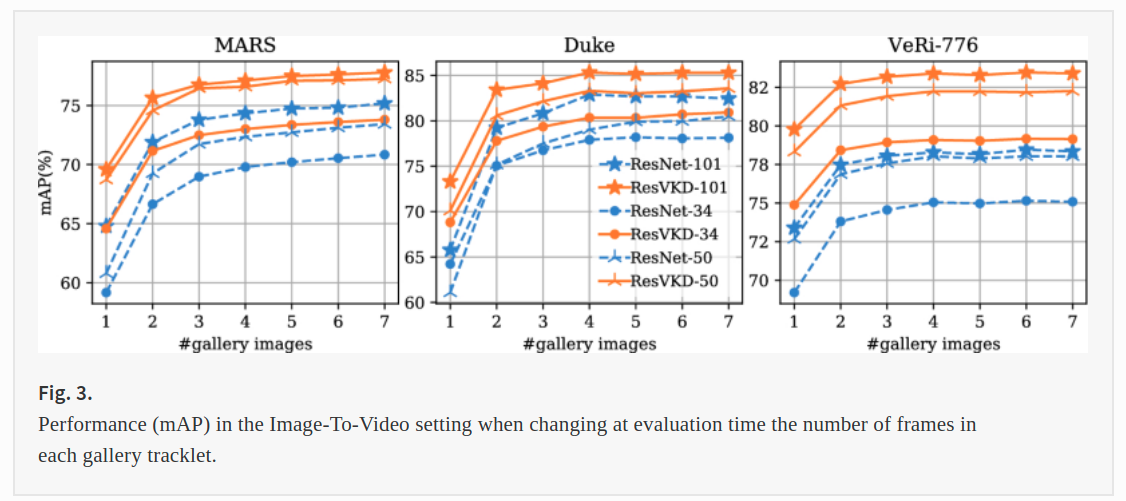As an additional proof, plots from Fig. 3 draw a comparison between models before and after distillation. VKD improves metrics considerably on all three dataset, as highlighted by the bias between the teachers and their corresponding students. Surprisingly, this often applies when comparing lighter students with deeper teachers: as an example, ResVKD-34 scores better than even ResNet-101 on VeRi-776, regardless of the number of images sampled for a gallery tracklet.

## Comparison with State-Of-The-Art

### Image-To-Video.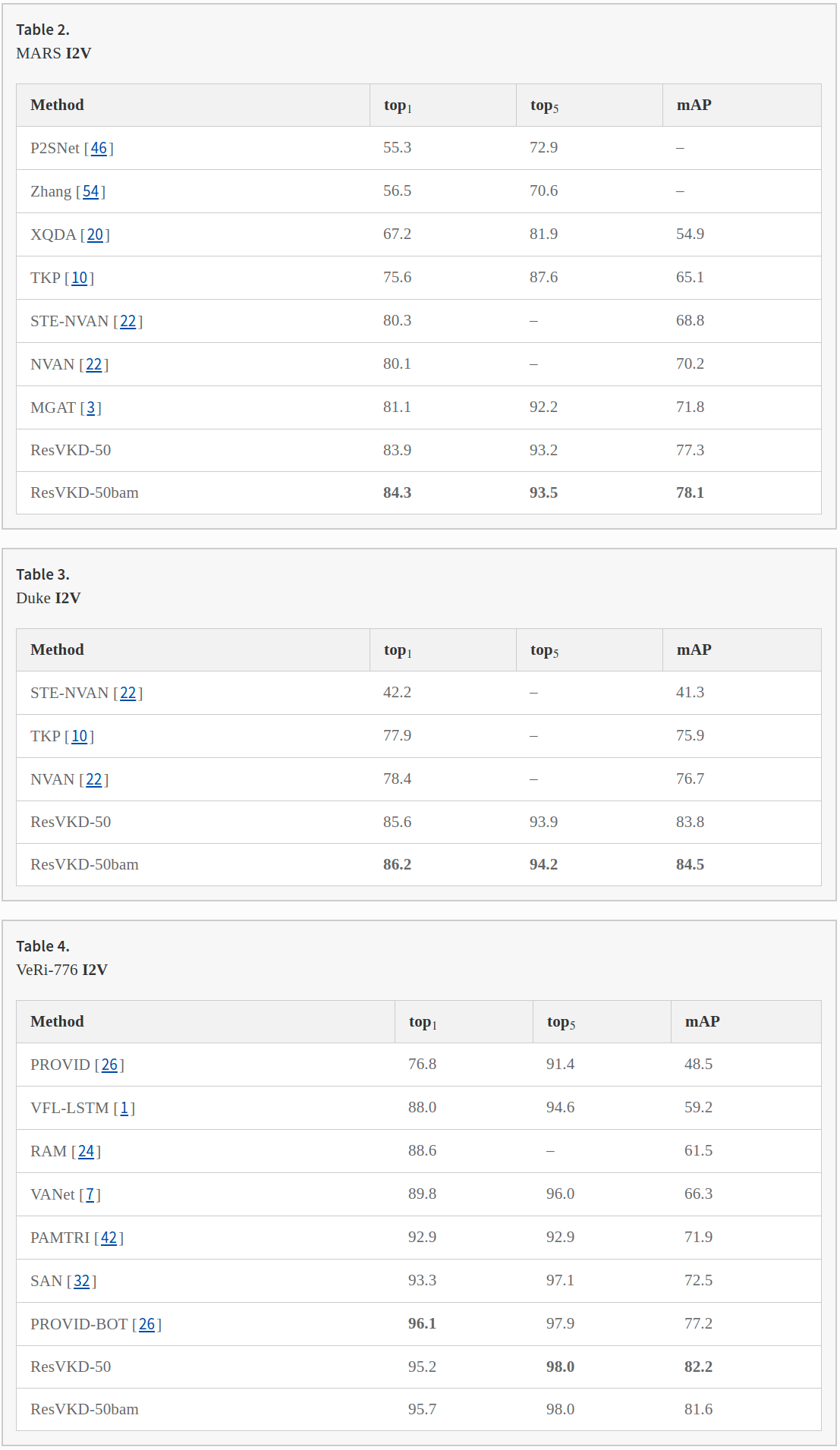Tables 2, 3 and 4 report a thorough comparison with current state-of-the-art (SOTA) methods, on MARS, Duke and VeRi-776 respectively. As common practice [3, 10, 32], we focus our analysis on ResNet-50, and in particular on its distilled variants ResVKD-50 and ResVKD-50bam. Our method clearly outperforms other competitors, with an increase in mAP w.r.t. top-scorers of 6.3% on MARS, 8.6% on Duke and 5% on VeRi-776. This results is totally in line with our goal of conferring robustness when just a single image is provided as query. In doing so, we do not make any task-specific assumption, thus rendering our proposal easily applicable to both person and vehicle Re-ID.

### Video-To-Video.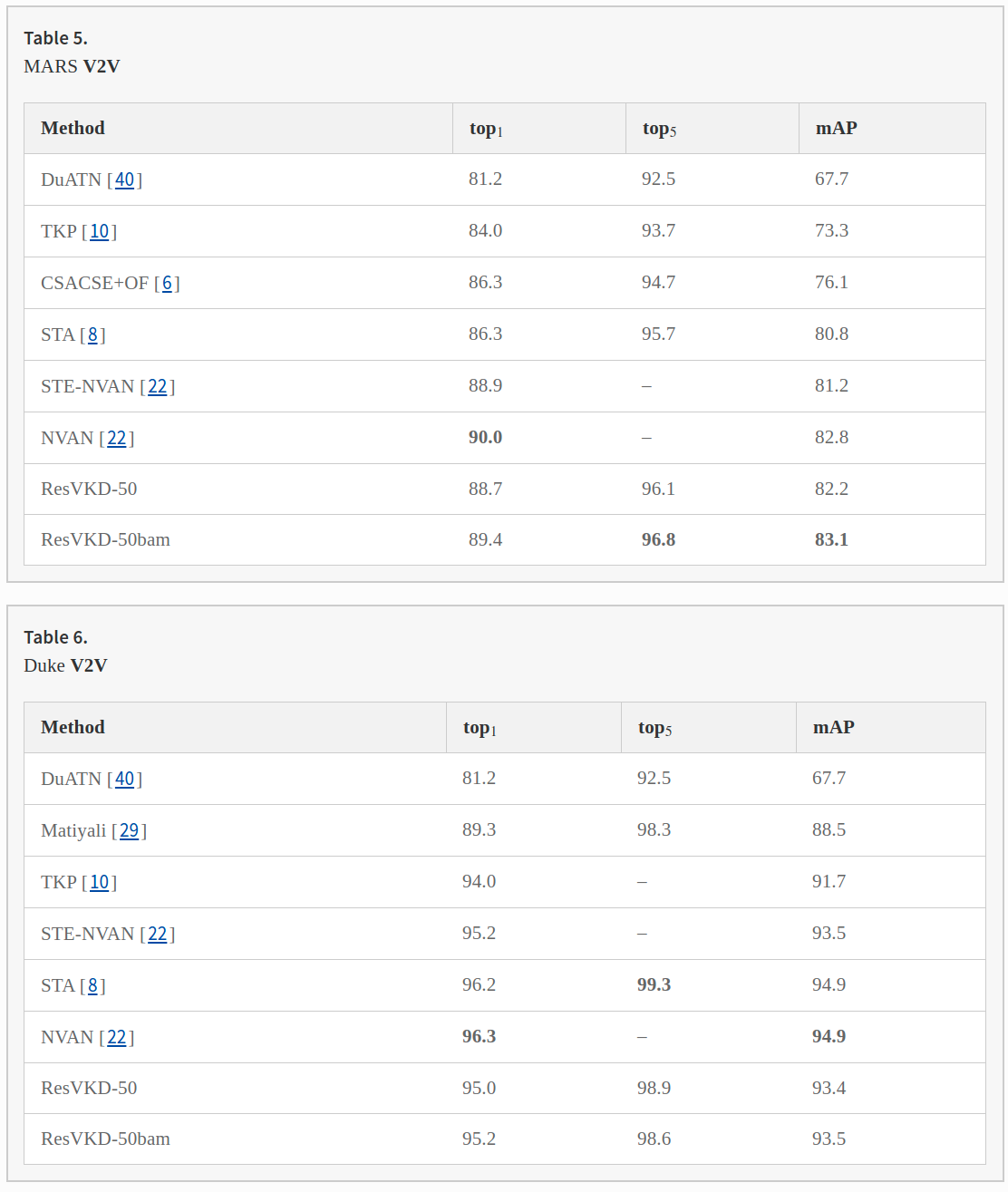Analogously, we conduct experiments on the V2V setting and report results in Table 5 (MARS) and Table 6 (Duke)4. Here, VKD yields the following results: on the one hand, on MARS it pushes a baseline architecture as ResVKD-50 close to NVAN and STE-NVAN , the latter being tailored for the V2V setting. Moreover – when exploiting spatial attention modules (ResVKD-50bam) – it establishes new SOTA results, suggesting that a positive transfer occurs when matching tracklets also. On the other hand, the same does not hold true for Duke, where exploiting video features as in STA  and NVAN appears rewarding. We leave the investigation of further improvements on V2V to future works. As of today, our proposals is the only one guaranteeing consistent and stable results under both I2V and V2V settings.

## Analysis on VKD

### In the Absence of Camera Information.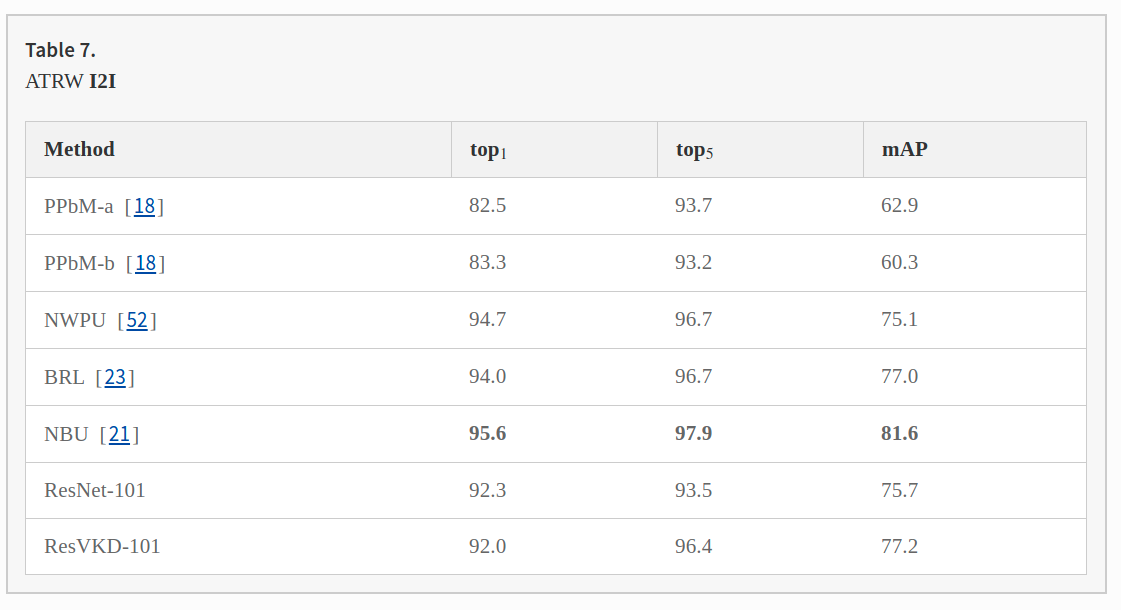### Distilling Viewpoints vs time.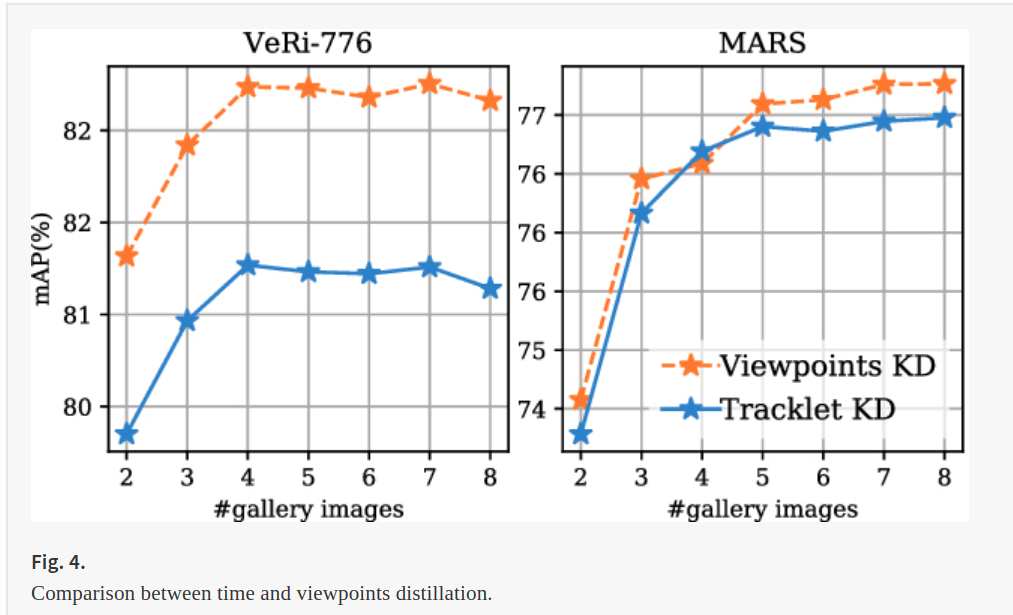### VKD Reduces the Camera Bias.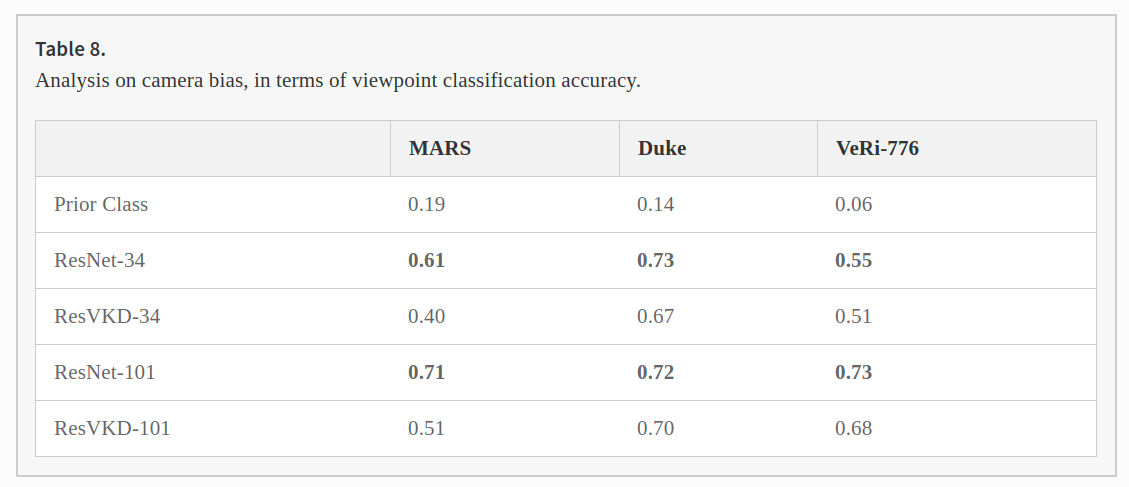### Can Performance of the Student be Obtained Without Distillation?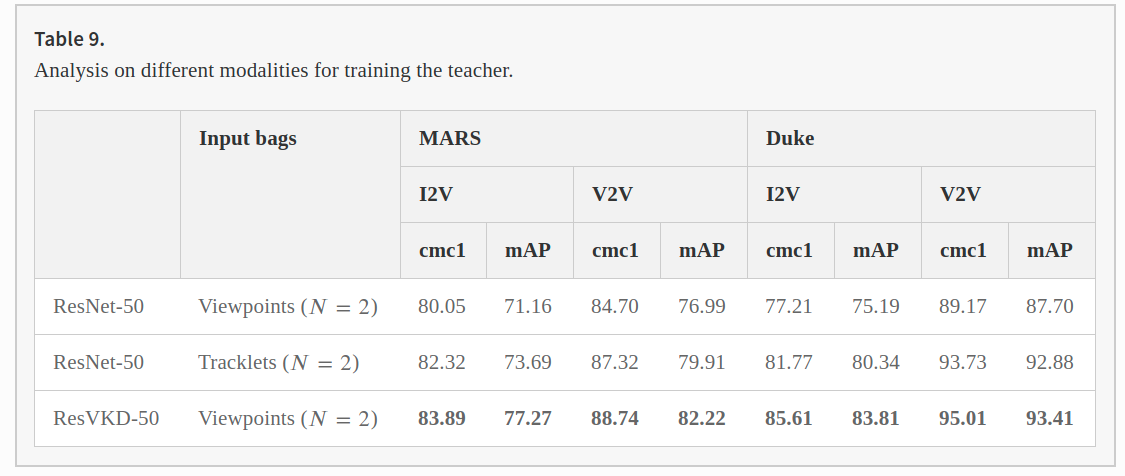### Student Explanation.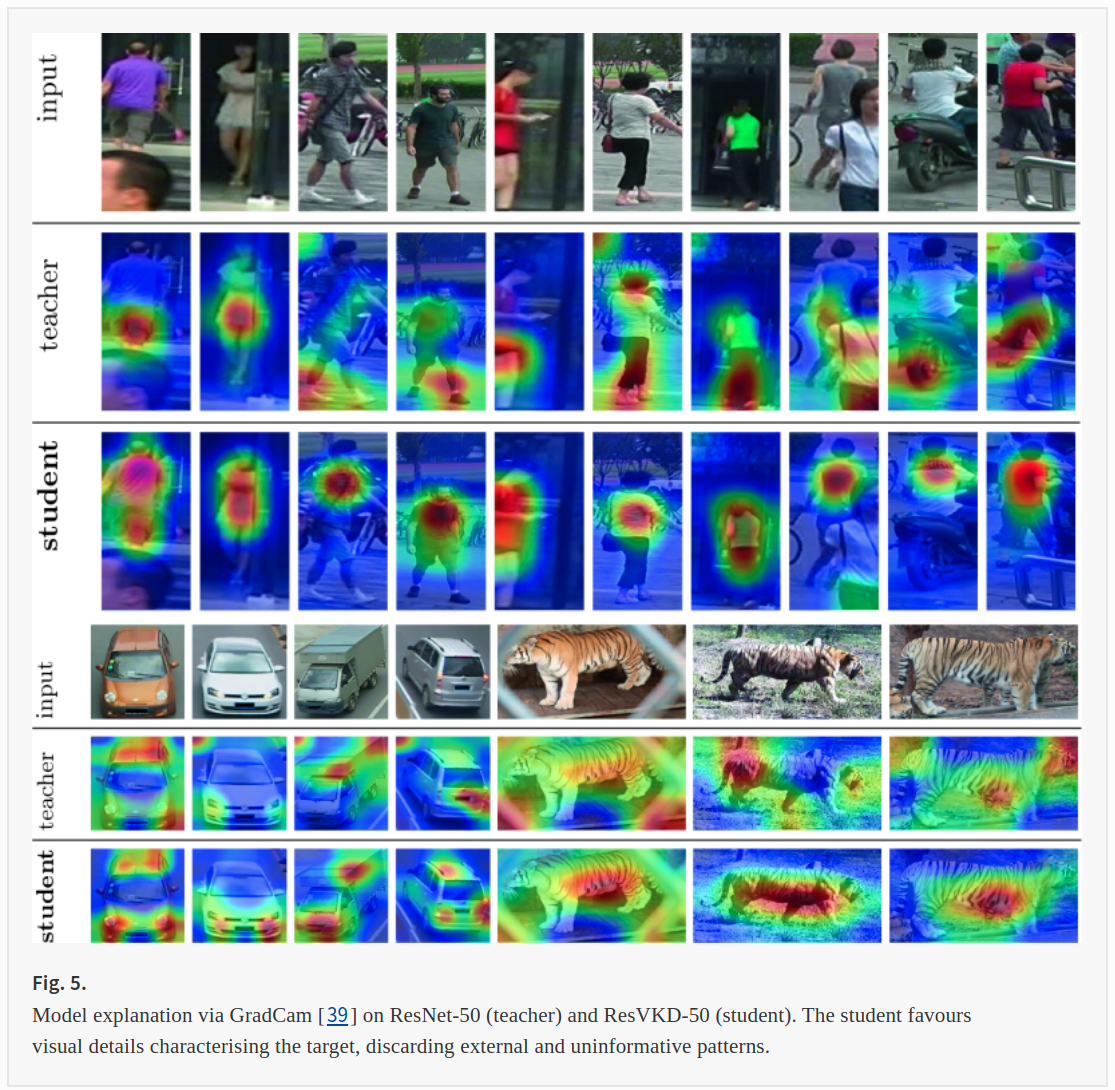### Cross-distillation.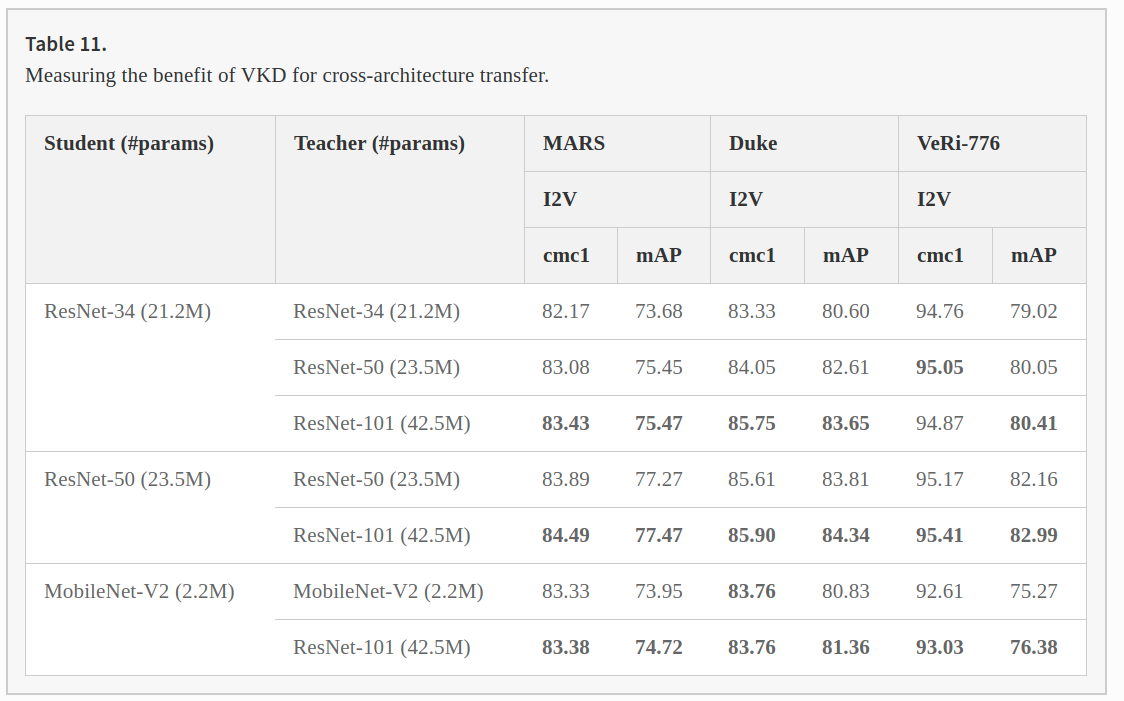### On the Impact of Loss Terms.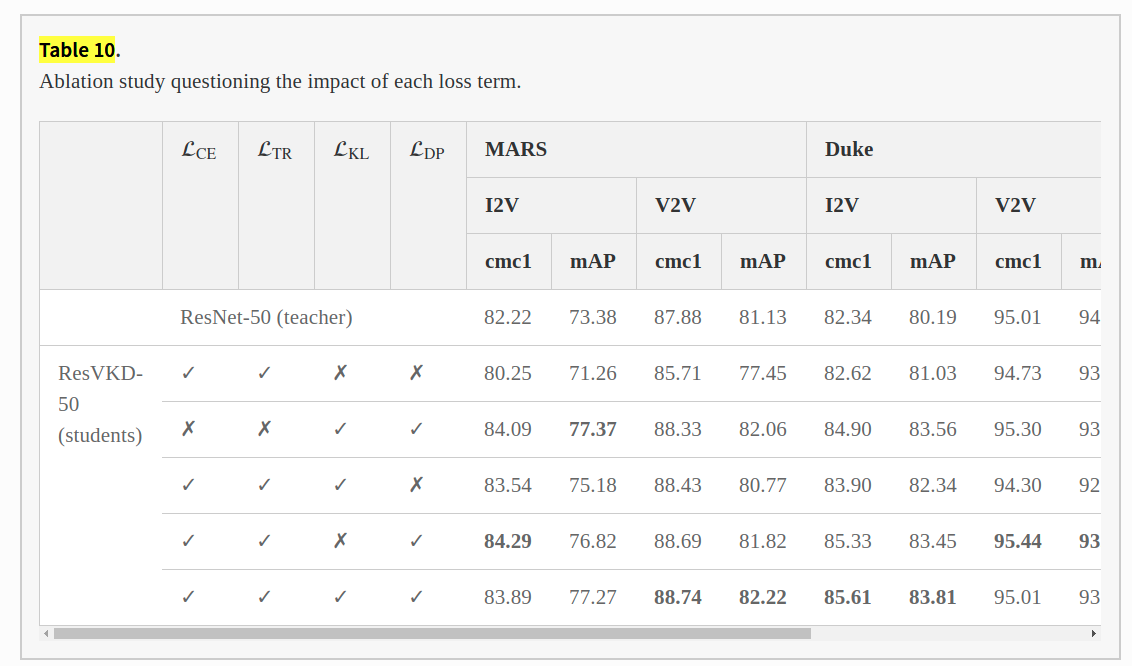# Conclusion